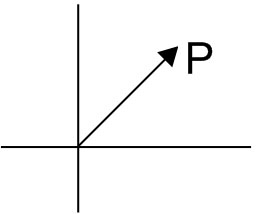#### If position vectors of A, B, C, D are respectivelyandthen Option 1)Option 2)Option 3) A,B,C are collinear Option 4) B,C,D are collinearAs we learnt

Position vector -

Let O be a fixed origin, then position vector of P is- whereinSinceSo thatSimilarlySo that ratios are equal. so they are parellalOption 1)Correct option

Option 2)Incorrect Option

Option 3)

A,B,C are collinear

Incorrect Option

Option 4)

B,C,D are collinear

Incorrect Option